# Comparable and Comparator Nuts and Bolts Nuts and

• Slides: 17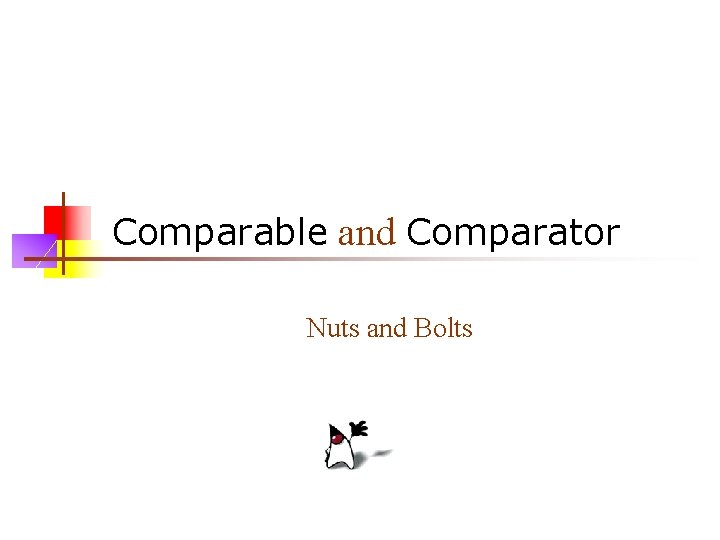Comparable and Comparator Nuts and BoltsNuts and bolts n Four methods underlie many of Java’s important Collection types: equals, compare and compare. To, and hash. Code n n n To put your own objects into a Collection, you need to ensure that these methods are defined properly Any collection with some sort of membership test uses equals (which, in many cases, defaults to ==) Any collection that depends on sorting requires larger/equal/smaller comparisons (compare or compare. To) Any collection that depends on hashing requires both equality testing and hash codes (equals and hash. Code) Any time you implement hash. Code, you must also implement equals Some of Java’s classes, such as String, already define all of these properly for you n For your own objects, you have to do it yourself 2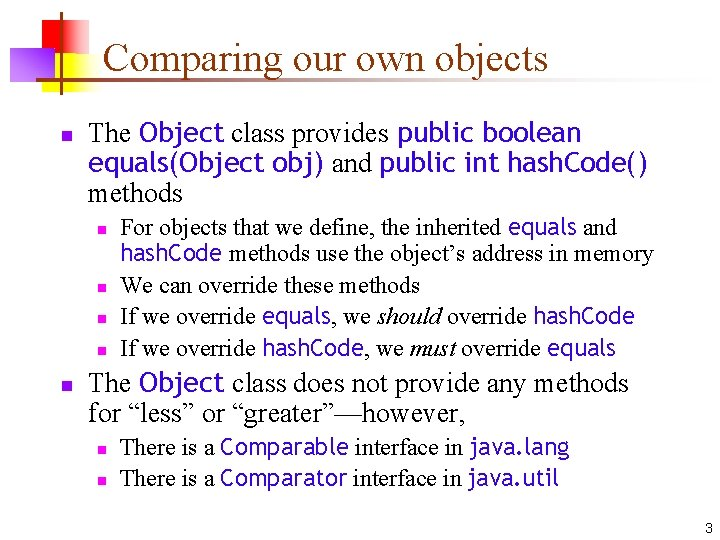Comparing our own objects n The Object class provides public boolean equals(Object obj) and public int hash. Code() methods n n n For objects that we define, the inherited equals and hash. Code methods use the object’s address in memory We can override these methods If we override equals, we should override hash. Code If we override hash. Code, we must override equals The Object class does not provide any methods for “less” or “greater”—however, n n There is a Comparable interface in java. lang There is a Comparator interface in java. util 3Outline of a Student class public class Student implements Comparable { public Student(String name, int score) {. . . } public int compare. To(Object o) throws Class. Cast. Exception {. . . } public static void main(String args[]) {. . . } } 4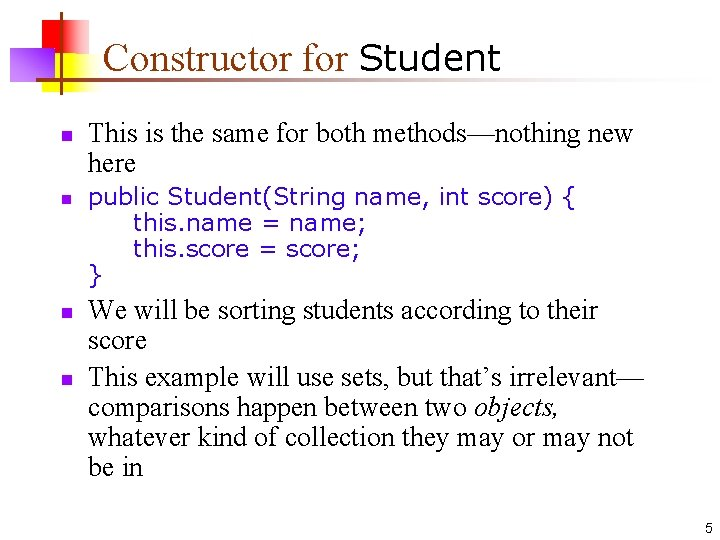Constructor for Student n n This is the same for both methods—nothing new here public Student(String name, int score) { this. name = name; this. score = score; } We will be sorting students according to their score This example will use sets, but that’s irrelevant— comparisons happen between two objects, whatever kind of collection they may or may not be in 5The main method, version 1 public static void main(String args[]) { Tree. Set<Student> set = new Tree. Set<Student>(); set. add(new } Student("Ann", 87)); Student("Bob", 83)); Student("Cat", 99)); Student("Dan", 25)); Student("Eve", 76)); Iterator<Student> iter = set. iterator(); while (iter. has. Next()) { Student s = iter. next(); System. out. println(s. name + " " + s. score); } 6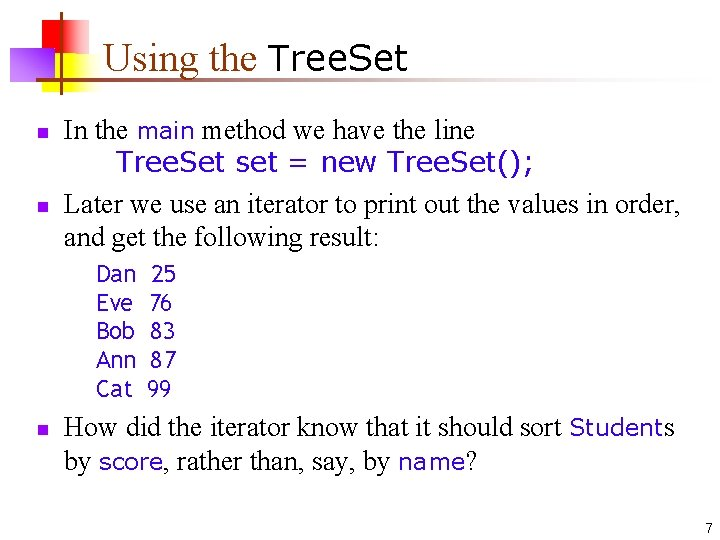Using the Tree. Set n n In the main method we have the line Tree. Set set = new Tree. Set(); Later we use an iterator to print out the values in order, and get the following result: Dan Eve Bob Ann Cat n 25 76 83 87 99 How did the iterator know that it should sort Students by score, rather than, say, by name? 7Implementing Comparable<T> n public class Student implements Comparable n This means it must implement the method public int compare. To(Object o) n n Notice that the parameter is an Object In order to implement this interface, our parameter must also be an Object, even if that’s not what we want public int compare. To(Object o) throws Class. Cast. Exception { if (o instanceof Student) return score - ((Student)o). score; else throw new Class. Cast. Exception("Not a Student!"); } A Class. Cast. Exception should be thrown if we are given a non. Student parameter 8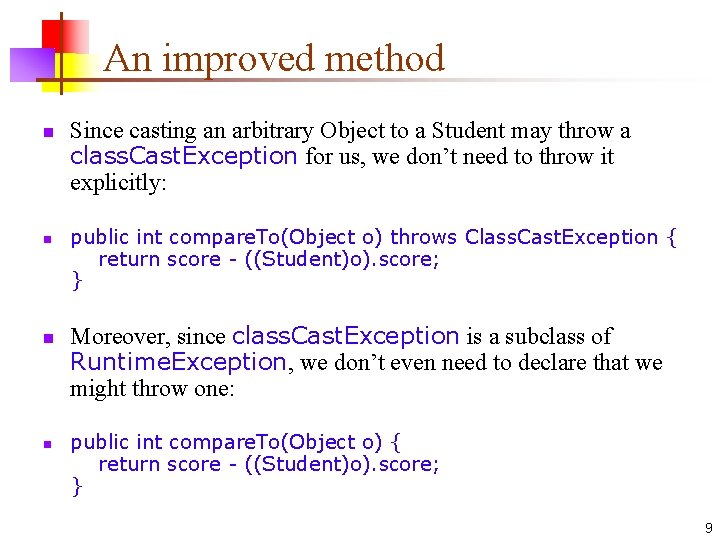An improved method n n Since casting an arbitrary Object to a Student may throw a class. Cast. Exception for us, we don’t need to throw it explicitly: public int compare. To(Object o) throws Class. Cast. Exception { return score - ((Student)o). score; } Moreover, since class. Cast. Exception is a subclass of Runtime. Exception, we don’t even need to declare that we might throw one: public int compare. To(Object o) { return score - ((Student)o). score; } 9Using a separate Comparator n In the program we just finished, Student implemented Comparable Therefore, it had a compare. To method n We could sort students only by their score n If we wanted to sort students another way, such as by name, we are out of luck Now we will put the comparison method in a separate class that implements Comparator instead of Comparable n n n This is more flexible (you can use a different Comparator to sort Students by name or by score), but it’s also clumsier Comparator is in java. util, not java. lang Comparable requires a definition of compare. To but Comparator requires a definition of compare Comparator also (sort of) requires equals 10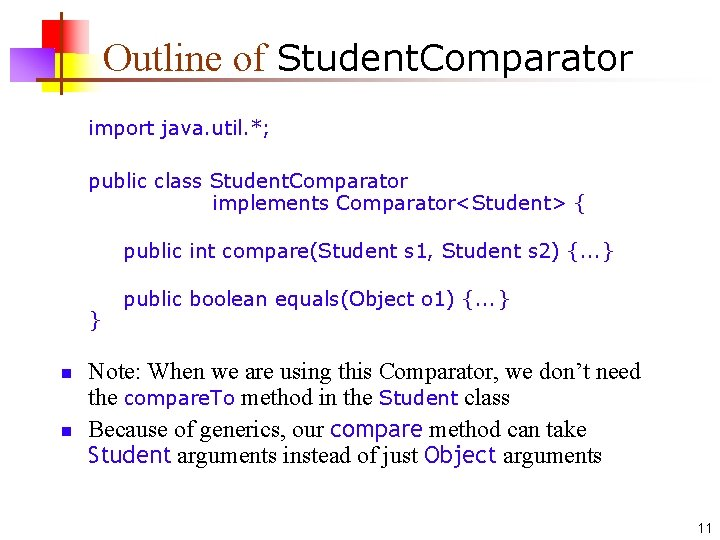Outline of Student. Comparator import java. util. *; public class Student. Comparator implements Comparator<Student> { public int compare(Student s 1, Student s 2) {. . . } } n n public boolean equals(Object o 1) {. . . } Note: When we are using this Comparator, we don’t need the compare. To method in the Student class Because of generics, our compare method can take Student arguments instead of just Object arguments 11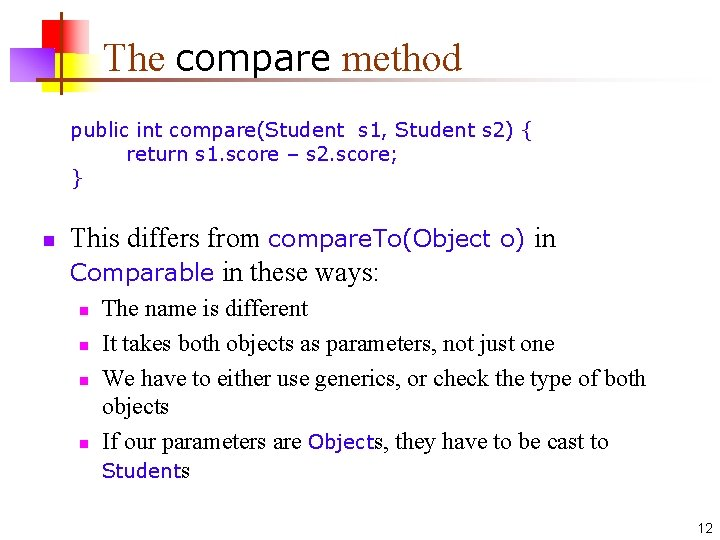The compare method public int compare(Student s 1, Student s 2) { return s 1. score – s 2. score; } n This differs from compare. To(Object o) in Comparable in these ways: n n The name is different It takes both objects as parameters, not just one We have to either use generics, or check the type of both objects If our parameters are Objects, they have to be cast to Students 12The some. Comparator. equals method n Ignore this method! n n This method is not used to compare two Students—it is used to compare two Comparators Even though it’s part of the Comparator interface, you don’t actually need to override it n n Implementing an interface requires you to have a definition for every method in the interface--so how can this be an exception? Because you do have a definition, inherited from Object ! In fact, it’s always safe to ignore this method The purpose is efficiency—you can replace one Comparator with an equal but faster one 13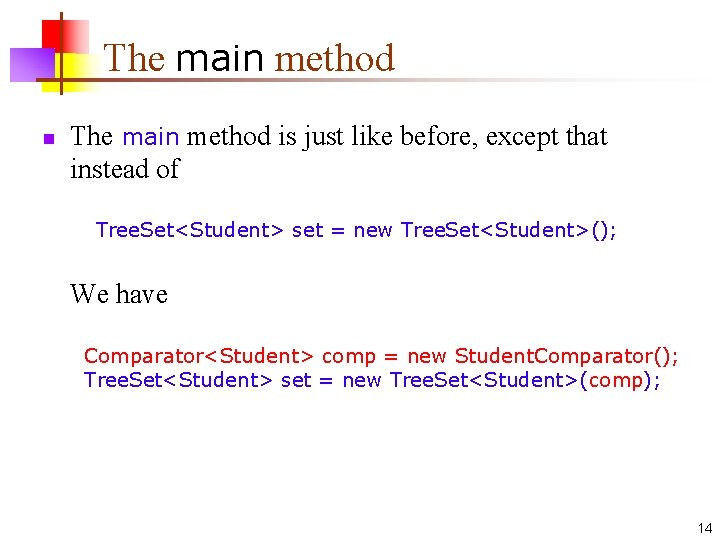The main method n The main method is just like before, except that instead of Tree. Set<Student> set = new Tree. Set<Student>(); We have Comparator<Student> comp = new Student. Comparator(); Tree. Set<Student> set = new Tree. Set<Student>(comp); 14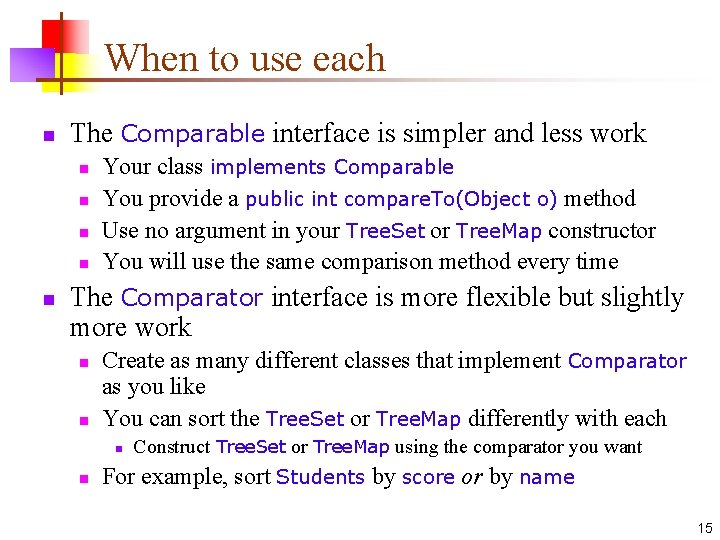When to use each n The Comparable interface is simpler and less work n n n Your class implements Comparable You provide a public int compare. To(Object o) method Use no argument in your Tree. Set or Tree. Map constructor You will use the same comparison method every time The Comparator interface is more flexible but slightly more work n n Create as many different classes that implement Comparator as you like You can sort the Tree. Set or Tree. Map differently with each n n Construct Tree. Set or Tree. Map using the comparator you want For example, sort Students by score or by name 15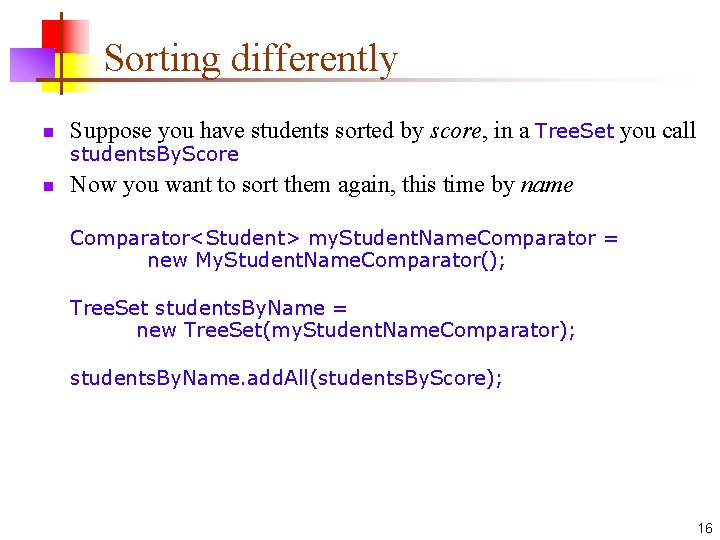Sorting differently n Suppose you have students sorted by score, in a Tree. Set you call n Now you want to sort them again, this time by name students. By. Score Comparator<Student> my. Student. Name. Comparator = new My. Student. Name. Comparator(); Tree. Set students. By. Name = new Tree. Set(my. Student. Name. Comparator); students. By. Name. add. All(students. By. Score); 16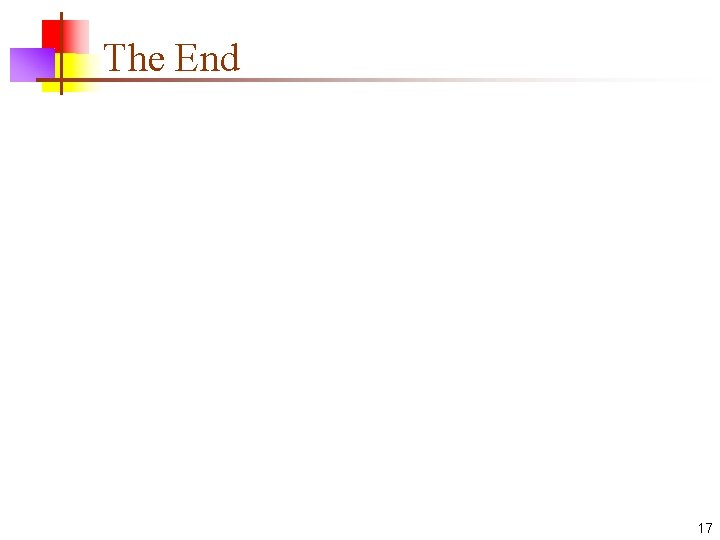The End 17